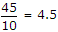# Electronics and Communication Engineering - Microprocessors

### Exercise :: Microprocessors - Section 4

21.

The signal in 8086 are in minimum mode when

 A. MN / Mx pin is tied to Vcc B. MN / Mx pin is grounded C. MN / MX pin is left open D. none of the above

Explanation:

No answer description available for this question. Let us discuss.

22.

If it is desired to tabulate number of students in a class in Fortran language, a proper variable name is

 A. ST B. STUD C. NSTUD D. STD

Explanation:

It is an integer. Hence N as first character.

23.

Let JCOKE = 20 and LPEPSI =15. Consider the statement `IF(2* JCOKE. LE. LPEPSI)JCOKE = JCOKE + 5 JCOKE = JCOKE + 3` The value of JCOKE after the execution of above statement will be

 A. 25 B. 23 C. 15 D. 17

Explanation:

Since 2 x J COKE = 40 and (LPEPSI) is 15 control goes to statement J COKE = J COKE + 3 and result is 23.

24.

In Pascal if I = 45, I div 10 * 10 =

 A. 45 B. 40 C. 0.45 D. 0.4

Explanation:→ 4 x 10 = 40.

25.

X COPY is an internal DOS command.

 A. True B. False